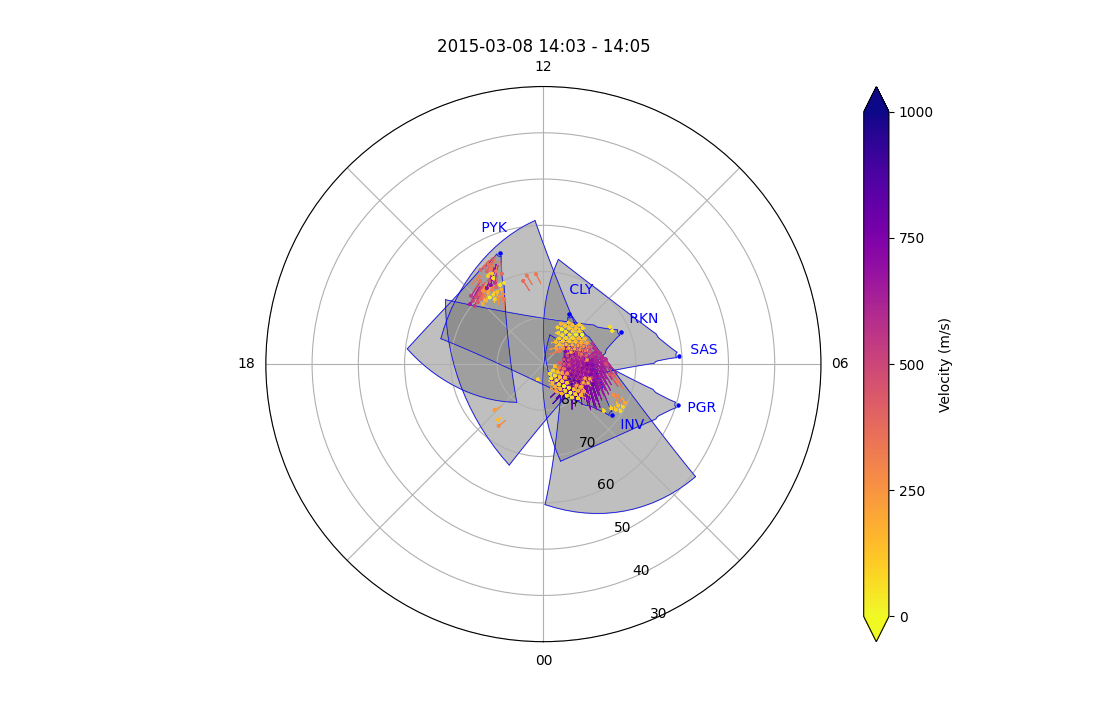# Grid plots

Grid plots are a way to visualise data from a GRID file of a SuperDARN radar(s). Please read RST documentation on how to process GRID files and GRD files from FITACF files.

Gridded parameters given in a grid file are:

Name parameter name
Line-of-sight Velocity `vec`
Power `pwr`
Spectral Width `wdt`

and a time or record number projected onto a polar format plot in AACGMv2 coordinates.

Currently, grid plots in pyDARN get geomagnetic positions of the gridded data in `mlon` and `mlat` from the GRID file, which uses AACGMv2 coordinates. In the future, pyDARN will also generate the geographic position of the data points, which will bring support for non-standard gridded vector layouts.

### Basic usage

pyDARN and pyplot need to be imported and the desired GRID file needs to be read in:

``````import matplotlib.pyplot as plt
import pydarn

file = "path/to/grid/file"

``````

With the grid data loaded as a list of dictionaries (`grid_data` variable in above example), you may now call the `plot_grid` method. Make sure you tell the method what time, in DateTime format, or record number (numbered from first recorded in file, counting from 0):

``````import datetime as dt

stime = dt.datetime(year, month, day, hour, minute)
gridplot = pydarn.Grid.plot_grid(grid_data, start_time=stime)
plt.show()

``````

In this example, the record at `stime` was plotted with the defaulted parameter, line-of-sight velocity, being gridded:You might have noticed that the variable `gridplot` in the examples above actually holds some information. This contains the AACGM latitude and longitude of the gridded vectors plotted, as well as the data. If you instead change `gridplot` to 3 separate variables, it will return the latitude, longitude, and data info into separate variables:

``````lats,lons,data=pydarn.Grid.plot_grid(grid_data, start_time=stime)
``````

In addition to the gridded line-of-sight velocity, you can choose one of two other data products to plot by setting `parameter=String name`:

Data product String name
Line of sight velocity (m/s) [Default] `vec`
Spectral width (m/s) `wdt`
Power (dB) `pwr`

Here is a list of all the current options than can be used with `plot_grid`

Option Action
record=(int) Record number to plot
start_time=(datetime.datetime) The start time of the record to plot
time_delta=(int) How close to the start time to be to the start time of the record
lowlat=(int) Control the lower latitude boundary of the plot (default 30/-30 AACGM lat)
fov=(bool) Boolean to show the Field-of-View of the radar(s)
cmap=matplotlib.cm A matplotlib color map object. Will override the pyDARN defaults for chosen parameter
zmin=(int) Minimum data value for colouring
zmax=(int) Maximum data value for colouring
colorbar=(bool) Set true to plot a colorbar (default: True)
colorbar_label=(string) Label for the colour bar (requires colorbar to be true)
title=(str) To add a title to the plot
radar_label=(str) To include a dot at radar location and label of 3 letter code
fov_color=(str) Fill color of fov
line_color=(str) Fill color of fov lines

As an example, the following code plots multiple radar Grid plot:

``````import matplotlib.pyplot as plt
import datetime as dt
import pydarn